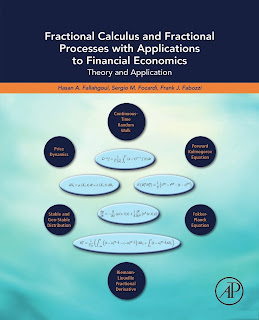CALCULUS MATHEMATICS

# Fractional Calculus and Fractional Processes with Applications to Financial Economics Theory and Application Free DownloadFractional Calculus and Fractional Processes with Applications to Financial Economics presents the theory and application of fractional calculus and fractional processes to financial data. Fractional calculus dates back to 1695 when Gottfried Wilhelm Leibniz first suggested the possibility of fractional derivatives. Research on fractional calculus started in full earnest in the second half of the twentieth century. The fractional paradigm applies not only to calculus, but also to stochastic processes, used in many applications in financial economics such as modelling volatility, interest rates, and modelling high-frequency data. The key features of fractional processes that make them interesting are long-range memory, path-dependence, non-Markovian properties, self-similarity, fractal paths, and anomalous diffusion behaviour. In this book, the authors discuss how fractional calculus and fractional processes are used in financial modelling and finance economic theory. It provides a practical guide that can be useful for students, researchers, and quantitative asset and risk managers interested in applying fractional calculus and fractional processes to asset pricing, financial time-series analysis, stochastic volatility modelling, and portfolio optimization.